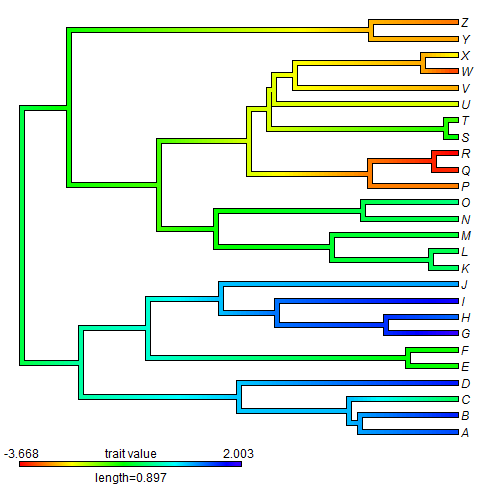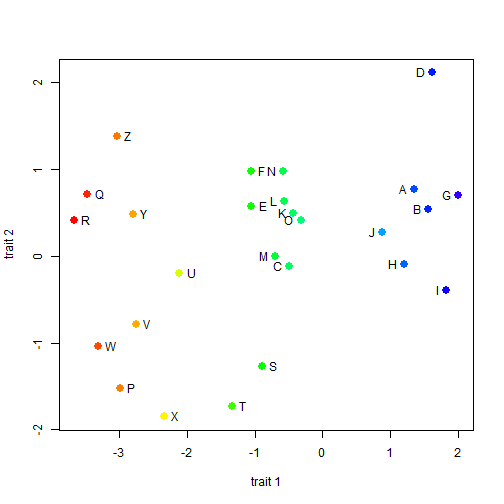## Wednesday, May 6, 2015

### How to get the colors of the tips in a plotted "contMap" object

Today I received an interesting question about the phytools function `contMap`. `contMap`, remember, is a function that maps the observed & reconstructed value of a continuous character on to the tree. The question is as follows:

“How can I recover the color codes that are used to paint the tips (or the last bit) of a phylogeny after a reconstruction with contMap? I have a character mapped on my phylogeny and I want to use the same tip colors in a scatter plot (first two components of a PCA in this case)… each species in the plot should have the same color as in the tree. I tried going through the code but I get lost at some point and can't figure out how the last bit of the terminal branches are indexed and how the color assigment is done…”

An object created by `contMap` is actually just the same object that is read & plotted by `plotSimmap`, which `plot.contMap` uses internally. The colors are encoded in the `"contMap"` element `cols`, and the corresponding indices for the colors mapped along each edge, in the object element `tree\$maps`.

To demo how we can pull the colors of all mapped species from this object, see the following demo:

``````library(phytools)
## simulate tree & data
tree<-pbtree(n=26,tip.label=LETTERS)
X<-fastBM(tree,nsim=2)
colnames(X)<-c("trait 1","trait 2")
## contMap for the first character only
obj<-contMap(tree,X[,1])
````````````## function to pull out the color of a given tip
foo<-function(obj,tip){
jj<-which(obj\$tree\$tip.label==tip)
kk<-which(obj\$tree\$edge[,2]==jj)
setNames(obj\$cols[names(obj\$tree\$maps[[kk]])[length(obj\$tree\$maps[[kk]])]],
NULL)
}
colors<-sapply(obj\$tree\$tip.label,foo,obj=obj)
colors
``````
``````##           A           B           C           D           E           F
## "#004BFFFF" "#0023FFFF" "#00FF5FFF" "#0018FFFF" "#0EFF00FF" "#10FF00FF"
##           G           H           I           J           K           L
## "#3000FFFF" "#0062FFFF" "#0F00FFFF" "#00A1FFFF" "#00FF64FF" "#00FF4DFF"
##           M           N           O           P           Q           R
## "#00FF33FF" "#00FF49FF" "#00FF79FF" "#FF7F00FF" "#FF2400FF" "#FF0300FF"
##           S           T           U           V           W           X
## "#00FF03FF" "#3EFF00FF" "#D9FF00FF" "#FFAE00FF" "#FF4A00FF" "#FFF500FF"
##           Y           Z
## "#FFA400FF" "#FF7800FF"
``````

OK, now how about using these to plot in a scatterplot? We can do that in our two dimensional simulated space:

``````plot(X,col=colors,pch=19,cex=1.5)
## just to position the labels a bit more nicely
ii<-X[,1]>(max(X[,1])+min(X[,1]))/2
text(X[ii,],rownames(X)[ii],pos=2)
text(X[!ii,],rownames(X)[!ii],pos=4)
``````That's all there is to it!